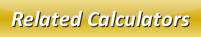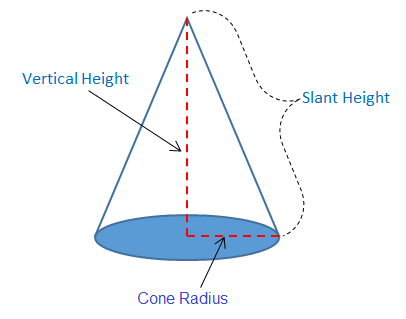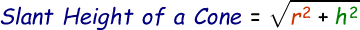# Slant Height of a Cone Calculator

Slant height of a cone is the length from top of the cone to down the side to the edge of the base as shown in the below figure. We can find the slant height of the cone when we know is vertical height and radius of the cone.Formula to calculate slant height of a cone is given by:where,
r = radius of the cone at base
h = vertical height from peak to base

Use our below online slant height of a cone calculator by entering the height and radius in the respective input boxes and then click calculate button to find the slant height of a cone with steps.

 Radius of the Cone (r): Height of the Cone (h): Slant Height of a Cone [Your Answer]:

Latest Calculator Release

Average Acceleration Calculator

Average acceleration is the object's change in speed for a specific given time period. ...

Free Fall Calculator

When an object falls into the ground due to planet's own gravitational force is known a...

Torque Calculator

Torque is nothing but a rotational force. In other words, the amount of force applied t...

Average Force Calculator

Average force can be explained as the amount of force exerted by the body moving at giv...

Angular Displacement Calculator

Angular displacement is the angle at which an object moves on a circular path. It is de...